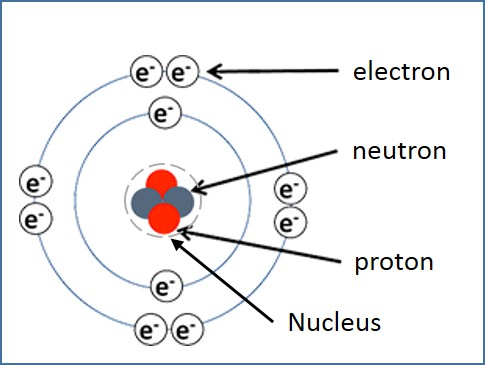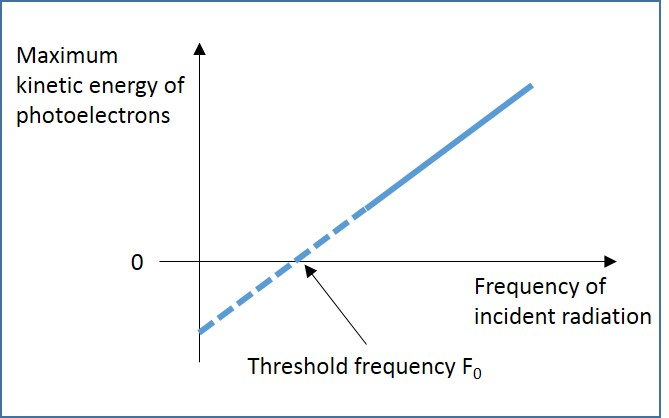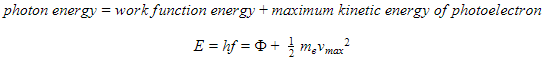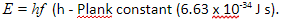# Photoelectric Effect

## Introduction

Protons, neutrons and electrons that move around the nucleus constitute an atom, as illustrated in Figure 1. Some of the electrons in a metal are free to move around. These electrons are called free electrons. To remove these free electrons from a metal, energy is required because they are held in the metal by the electrostatic attraction of the positively charged nuclei. The electron must be given energy in order to escape from the surface of a metal. If this energy is in the form of light energy, then this phenomenon is called photoelectric emission.Figure 1: Constituents of the Atom

A. Photoelectric Effect

Photoelectric emission is defined as the release of electrons from the surface of a metal when electromagnetic radiation is incident on its surface. The electrons that are released in the photoelectric emission are called photoelectrons. An experiment shown in Figure 2 is used to investigate the energy level of the photoelectrons.Figure 2: Investigation of Photoelectrons

The experiment can be performed using ultraviolet radiation of different frequencies. When the maximum kinetic energy of the photoelectrons is plotted against the frequency of the radiation, the following graph is obtained:Figure 3: Kinetic Energy of Photoelectron vs. Frequency of Radiation

B. Work Function Energy

The minimum amount of energy necessary for an electron to escape from the source is called work function energy Ф.

C. Threshold Frequency

Photoelectric emission takes place only if the frequency of the incident radiation is above a certain minimum value called the threshold frequency (fo).

D. Einstein’s Theory of Photoelectric Emission

The German physicist Max Plank suggested that the energy carried by electromagnetic radiation might exist as discrete packets called quanta. The energy (E) carried in each quantum is given by the following equation:According to Albert Einstein’s theory of quantised energy, light energy consists of a stream of energy packets called photons. A photon is defined as a quantum of energy when the energy is in the form of electromagnetic radiation. Einstein derived the photoelectric equation by using the principle of conservation of energy.E. Summary

• The electron must be given energy in order to escape from the surface of a metal. If this energy is in the form of light energy, then the phenomenon is called photoelectric emission.
• Photoelectric emission is defined as the release of electrons from the surface of a metal when electromagnetic radiation is incident on its surface.
• The electrons that are released in the photoelectric emission are called photoelectrons.
• The minimum amount of energy necessary for an electron to escape from the source is called the work function energy Ф.
• Photoelectric emission takes place only if the frequency of the incident radiation is above a certain minimum value called the threshold frequency (fo).
• Energy carried in each quantum,• A photon is defined as a quantum of energy when the energy is in the form of electromagnetic radiation.